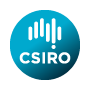# Software from Alan J. Miller

 Index Applied statistics algorithms Quadruple precision code (F) Quadruple precision Random Toms

# Miscellaneous TOMS (& CACM) algorithms

N.B. I have been asked to provide a link to the copyright policy of the ACM. Loosely paraphrased, this allows use, and modification, of the TOMS algorithms for most non-commercial purposes. It also emphasizes that the ACM accepts no responsibility for the accuracy of the code.

• cacm125.f90. Calculate ordinates and weights for Gaussian integration.
• cacm395.f90. Calculate 2-tailed probabilities from Student's t distribution.
• cacm396.f90 calculates quantiles of the t-distribution. These are translations of Geoff Hill's algol code; Geoff was acting chief of CSIRO Division of Mathematical Statistics at one time. There is also a test program which may be useful test395.f90.
• cpoly.f90 This is the classic Jenkins & Traub algorithm (CACM 419) for the solution of complex polynomials. q_cpoly.f90 is a version in quadruple precision.
• rpoly.f90 TOMS algorithm 493 for the solution of polynomial equations with real coefficients. q_rpoly.f90 is a version in quadruple precision.
• toms513.f90 In situ transpose of an MxN matrix.
• toms519.f90 Kolmogorov-Smirnov probabilities.
• toms530.f90 Eigenvalues & vectors of real skew-symmetric matrices (i.e. a(i,j) = -a(j,i) ), and of symmetric matrices with zero diagonal elements.
• toms533.zip A simple package for the solution of sparse linear equations. It is even shorter and simpler in Fortran 90 as the user does not supply work arrays. The zipped file (use PKUNZIP or WinZip to access the contents) contains two demo programs. The second of these contained an error in the F77 version, and the solution vector was completely wrong.
• toms615.f90 Fitting linear regression models by minimizing the sum of absolute deviations. i.e. using the L_1 norm. There are also, a short test program:
• test615.f90 A data file for the test program:
• test615.dat and a file showing the output:
• test615.out
• toms639.f90 Integration from zero to infinity of oscillating functions. Includes driver program.
• toms642.zip Fit a cubic spline to noisy data using generalized cross-validation. Data may be unevenly spaced, and have different weights. Bayesian point error estimates are generated.
• fexact.zip (TOMS 643) Fisher's exact test for two-way contingency tables. This is a zipped file including a driver, data sets, and output. Use pkunzip or winzip to unpack the files.
• toms644.zip Bessel and Airy functions for complex arguments. This is a zipped file including test programs. Use pkunzip or winzip to unpack the files.
• toms654 The incomplete gamma function and its inverse. Test program: t_incgam.f90
• toms660.f90 Quadratic spline interpolation of a bivariate function defined by irregularly spaced data.
• toms661.f90 Quadratic spline interpolation of a trivariate function defined by irregularly spaced data.
• toms655.zip IQPACK (Interpolation / Quadrature). Calculates nodes and weights for 8 types of Gaussian quadrature (Legendre, Chebyshev, Hermite, rational polynomial, etc.).
• toms667.f90 Global minimization using a stochastic algorithm, with 37 test problems test667.f90. Many of the test problems require hundreds of thousands of function evaluations. See also global.f90 below in the miscellaneous code section.
• toms668.f90 The file for r_hyperg.f90 contains TOMS algorithm 668 for generating random values from the hypergeometric distribution.
• toms683.f90 The exponential integral En(x) for complex argument x.
• toms703.f90 A package for the solution of systems of ordinary differential equations: DY/DT = F(Y,T). There is also a test program: test703.f90
• toms707.f90 Confluent hypergeometric function for complex arguments.
• toms715.zip Special functions by W.J. Cody and others. Particularly Bessel functions, but also the gamma, normal distribution, error, Dawson and psi functions.
• toms725.f90 Multivariate normal integrals for dimension up to 20.
• toms726.zip Walter Gautschi's package for generating orthogonal polynomials and Gauss-type quadrature rules. The zip file contains a double precision, and most of the single precision code, plus the set of 11 test programs and Gautschi's output.
• toms751.f90 Renka's TRIPACK package for constrained 2D Delaunay triangulation, including test program (with built-in data) and output files.
• toms757.zip Code for computing some uncommon special functions, including Abramowitz functions, Airy function variants, integrals of zero-order Bessel functions, Debye functions, Struve functions, Synchrotron radiation and transport functions, Lobachevski and Stromgen integrals, etc. A test program is included. This is a zip file.
• toms760.f90 Bicubic interpolation from a rectangular grid of data. There is also a test program: test760.f90
• toms766.zip Code for computing Pade'-Hermite and simultaneous Pade' approximants, plus two driver programs.
• toms778.zip This subroutine solves bound-constrained optimization problems by using the compact formula of the limited memory BFGS updates.
• tensolve.zip This contains both TOMS 739 (UNCMIN) for unconstrained minimization, and TOMS 768 (TENSOLVE) for the solution of sets of non-linear equations.
• toms790.zip Cubic interpolation of scattered data in two dimensions with continuous second derivatives.
• toms796.zip This package is for the numerical inversion of Laplace transforms. The original was in very poor Fortran 77 which used many non-standard features, and it contained many bugs. Each compiler I tried gave different errors, and then different results when the errors were corrected. This version is in standard Fortran and gives reproducible results.
• toms813.zip This package is for local minimization, with or without convex constraints. It requires the user to supply first derivatives. This version is in standard Fortran. It uses the so-called spectral projected gradient (SPG) method.
• toms819.zip This is for the computation of the Airy functions Ai(z) and Bi(z) and their derivatives for complex arguments.